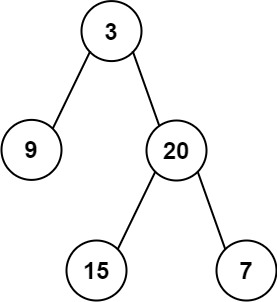# GeetCode Hub

Given two integer arrays `inorder` and `postorder` where `inorder` is the inorder traversal of a binary tree and `postorder` is the postorder traversal of the same tree, construct and return the binary tree.

Example 1:```Input: inorder = [9,3,15,20,7], postorder = [9,15,7,20,3]
Output: [3,9,20,null,null,15,7]
```

Example 2:

```Input: inorder = [-1], postorder = [-1]
Output: [-1]
```

Constraints:

• `1 <= inorder.length <= 3000`
• `postorder.length == inorder.length`
• `-3000 <= inorder[i], postorder[i] <= 3000`
• `inorder` and `postorder` consist of unique values.
• Each value of `postorder` also appears in `inorder`.
• `inorder` is guaranteed to be the inorder traversal of the tree.
• `postorder` is guaranteed to be the postorder traversal of the tree.

/** * Definition for a binary tree node. * public class TreeNode { * int val; * TreeNode left; * TreeNode right; * TreeNode() {} * TreeNode(int val) { this.val = val; } * TreeNode(int val, TreeNode left, TreeNode right) { * this.val = val; * this.left = left; * this.right = right; * } * } */ class Solution { public TreeNode buildTree(int[] inorder, int[] postorder) { } }